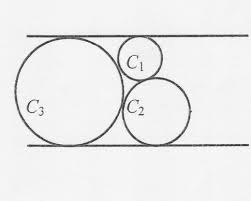# Three externally touching circles tangent to two paralle lines by HCR

Geometry Level 5Three externally touching circles C1, C2 & C3 are tangent to two parallel lines (as shown in the figure above). The radii of circles C1 & C2 are 6 & 12 units respectively. If the distance between the parallel tangent lines is a√b where a & b are positive integers (b is square free) find out the value of a+b

×